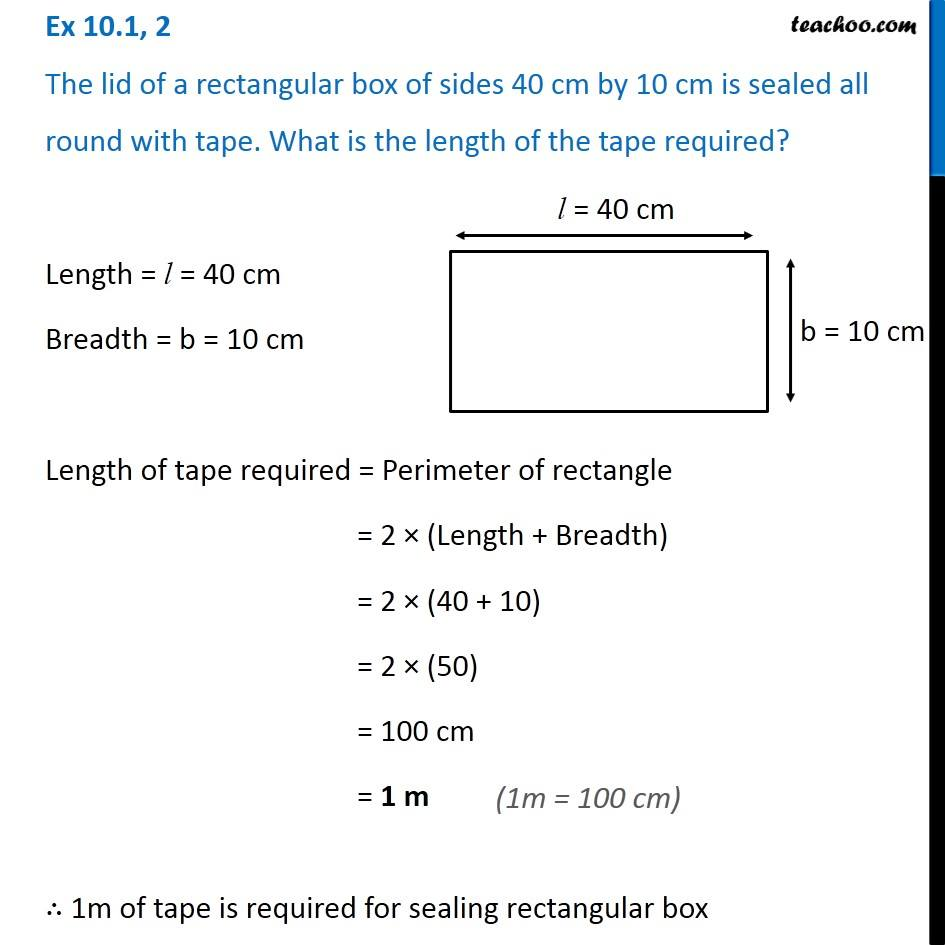Perimeter of Rectangle

Chapter 10 Class 6 Mensuration
Concept wiseGet live Maths 1-on-1 Classs - Class 6 to 12

### Transcript

Ex 10.1, 2 The lid of a rectangular box of sides 40 cm by 10 cm is sealed all round with tape. What is the length of the tape required? Length = l = 40 cm Breadth = b = 10 cm Length of tape required = Perimeter of rectangle = 2 × (Length + Breadth) = 2 × (40 + 10) = 2 × (50) = 100 cm = 1 m (1m = 100 cm) ∴ 1m of tape is required for sealing rectangular box